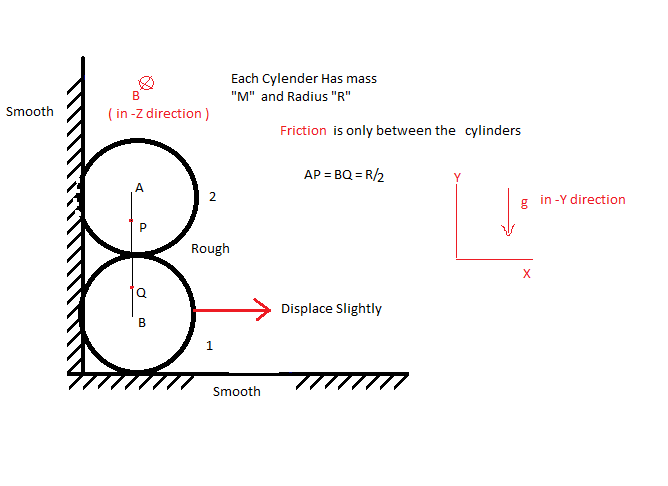# Mechanics + Magnetism = Mechatism 3As shown in figure there is Two Identical Conducting Hollow Cylinders each of mass M and Radius R . And there is sufficiently Friction between two the cylinders only.

Now If Lower cylinder is displace slightly to the rightward direction .And If Maximum velocity of lower cylinder is ${ V }_{ 1,max }$ .

Assume that whole system is placed in uniform magnetic field of strength B . which is perpendicular to the plane of cylinders ( - Z direction )

And If Magnitude of Motional EMF develop between the point P and Q just before the lower cylinder reaches it's maximum velocity is ${ E }_{ pq }$.

Then Find The value of $\displaystyle{\cfrac { BR({ V }_{ 1,max }) }{ { E }_{ pq } }}$.